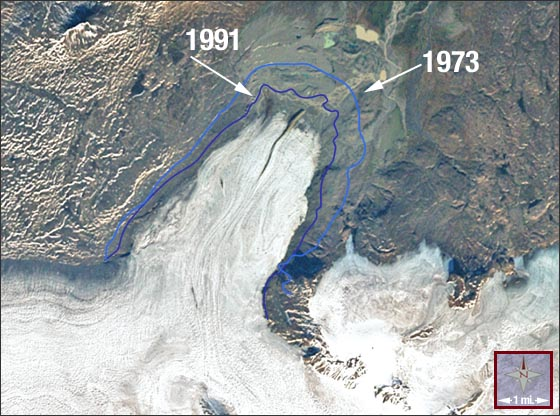## Glacier Retreat

Examine a true color satellite image to determine the volume of ice lost in the Eyjabakkajökull Glacier between 1973 and 1991.

### Mini LessonThe Eyjabakkajökull Glacier is an outlet glacier of the Vatnajökull ice cap in Iceland. It has been retreating since a major surge occurred in 1973. This true-color Landsat-7 image shows the glacier terminus in September 2000. The light- and dark blue outlines show the terminus extent in 1973 and 1991, respectively.

1. Using a metric ruler, and the conversion 1 mile = 1.61 kilometers, what is the scale of the image in meters per millimeter?
2. How many kilometers did the glacier retreat between A) 1973 and 1991? B) 1991 and 2000?
3. From your answers to Problem 2, what is the average rate of retreat in kilometers per year between A) 1973-1991, and B) 1991 to 2000? C) Is the retreat of the glacier speeding up or slowing down?
4. Assume that the height of the glacier is 1000 meters. About what volume of ice has been lost between 1973 and 1991 in cubic kilometers, assuming that the missing ice is shaped like a wall?

Sources:

1. Space Math http://spacemath.gsfc.nasa.gov
2. Landsat Math https://landsat.gsfc.nasa.gov/landsat-math-2/